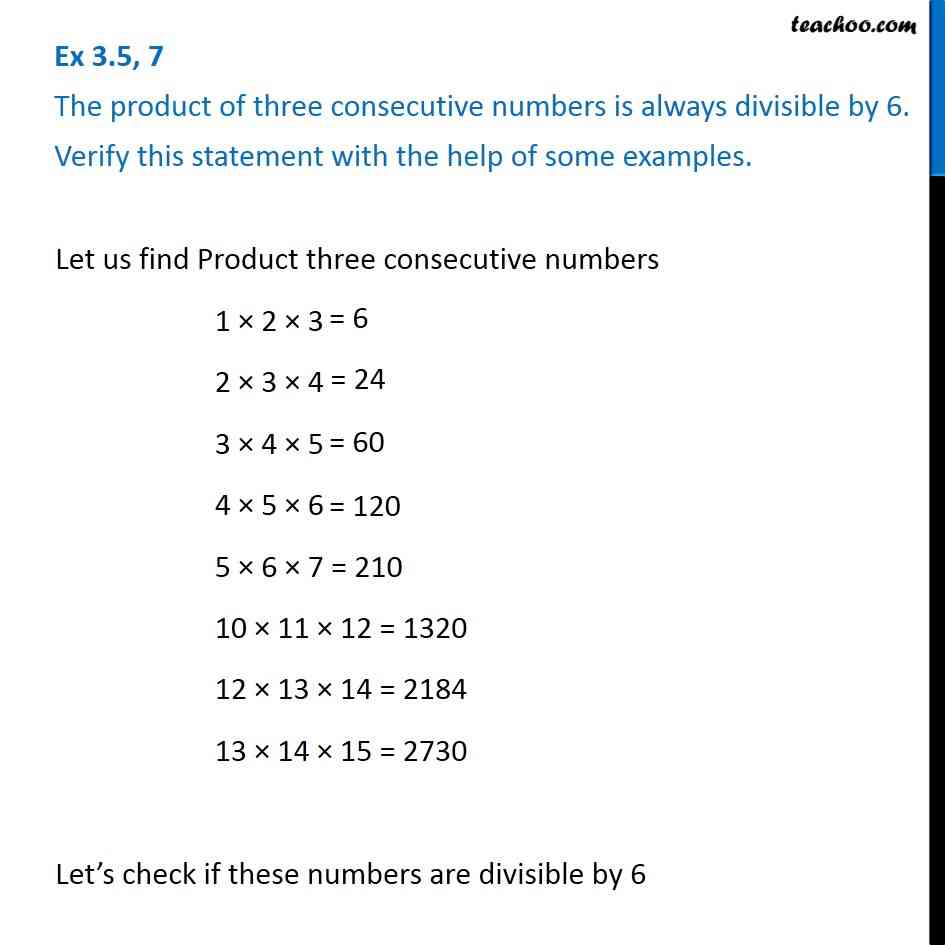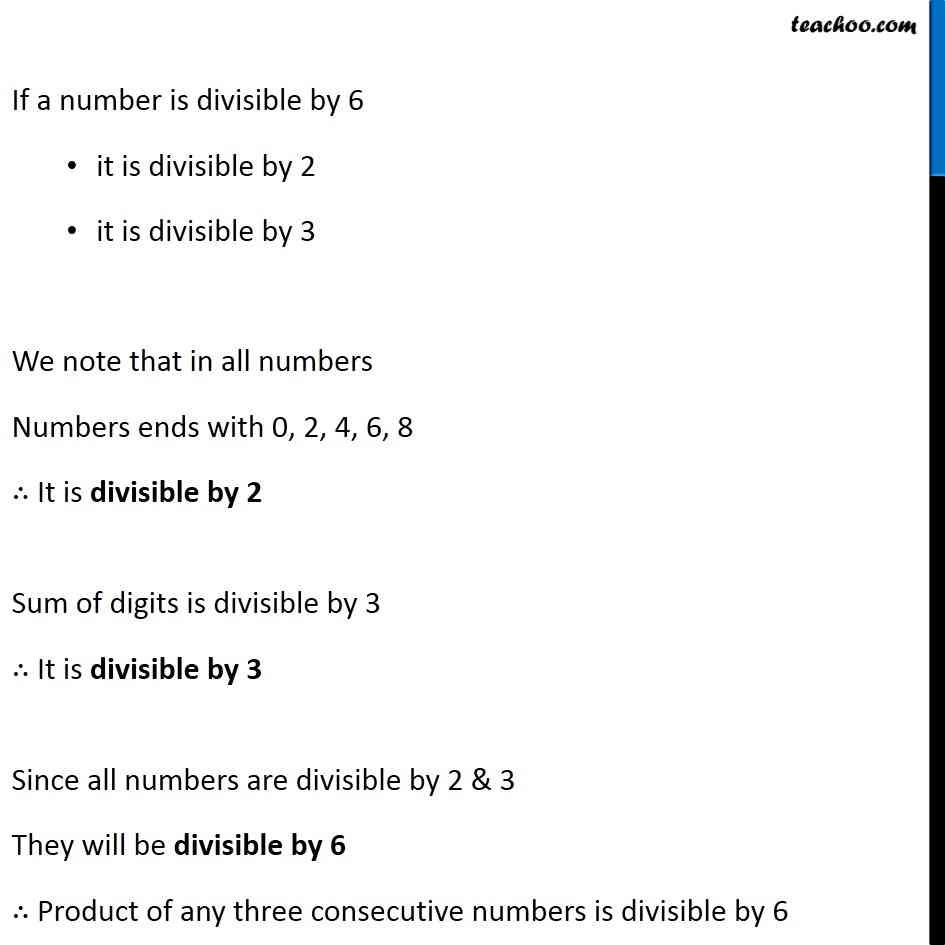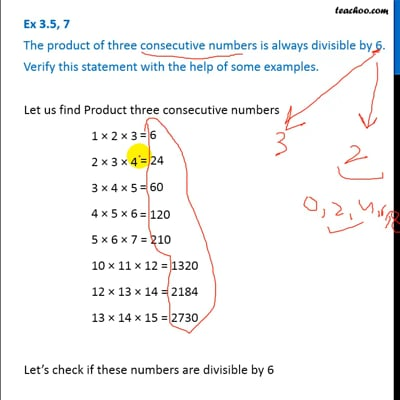Ex 3.5

Chapter 3 Class 6 Playing with Numbers
Serial order wiseThis video is only available for Teachoo black users

### Transcript

Ex 3.5, 7 The product of three consecutive numbers is always divisible by 6. Verify this statement with the help of some examples.Let us find Product three consecutive numbers 1 × 2 × 3 2 × 3 × 4 3 × 4 × 5 4 × 5 × 6 5 × 6 × 7 = 210 10 × 11 × 12 = 1320 12 × 13 × 14 = 2184 13 × 14 × 15 = 2730 Let’s check if these numbers are divisible by 6 If a number is divisible by 6 it is divisible by 2 it is divisible by 3 We note that in all numbers Numbers ends with 0, 2, 4, 6, 8 ∴ It is divisible by 2 Sum of digits is divisible by 3 ∴ It is divisible by 3 Since all numbers are divisible by 2 & 3 They will be divisible by 6 ∴ Product of any three consecutive numbers is divisible by 6